Next: 5 Simulation Up: 4.3 Physical Parameters Previous: 4.3.1 Effective Mass and Band Edge Energy

4.3.2 Mobility

Typical measured v(E) characteristics of III­V bulk material exhibit a local maximum as shown in  Figure 2.4. Simulations with a v(E) characteristics including a local maximum revealed low impact on the results of HEMTs but very unstable convergence in comparison to a v(E) monotonous characteristics like that of silicon.

The minor impact of the local maximum is because the electrons under the gate are in saturation for almost all relevant bias voltages of HEMTs which will be shown in Sections 5.3.1 and 5.3.2. The local maximum is obtained only for a certain range of electric fields between the constant mobility and the saturation velocity regime. Therefore, it has to be expected that this electric field is reached only in very small areas of the current path. On the other hand, the spatial velocity overshoot (described later in this section) and the saturation velocity (described in Section 2.1) of electrons in quantum wells is not known exactly. Thus, one can assume that the error made by neglecting the static velocity overshoot is small compared to uncertainties in the saturation velocity and spatial velocity overshoot.

In addition, convergence is usually poor if the thermionic field emission interface model (i. e. including tunneling) and a local maximum in the v(E) characteristics are combined. Therefore, the v(E) characteristics with a local maximum was not used further and a silicon like characteristics was assumed. The DD mobility for electrons and holes are modeled by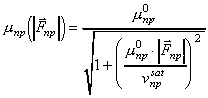, (44)

where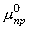are the zero-field mobility,are the saturation velocity and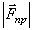are the magnitude of the driving force of electrons and holes, respectively. The driving forces are defined by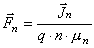and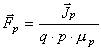. (45)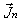and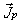are given by (19) and (20), respectively. If the DD model is considered, i. e. the carrier temperatures are constant, the driving forces for electrons and holes read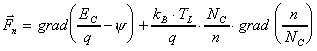(46)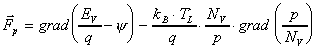, (47)

respectively.

For the HD model the mobility is modeled as a function of the carrier temperature Tnp: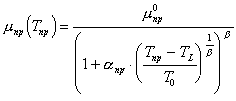. (48)

The temperatures are scaled by some arbitrary temperature T0. Initially (48) was developed by Haensch for silicon  where b = 1. The principal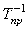dependence does not hold for III­V semiconductors. Monte Carlo simulations reveal decay ranges between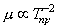and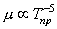[50, 51]. The parameter b can be used to model the decay of mobility for moderate carrier temperatures. For Tn = 0 and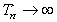the mobility is independent of b.

Considering energy balance, using a local approximation, and (25) the energy transport equation (21) and (22) reduce to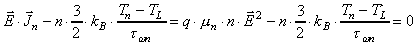(49)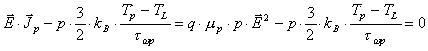(50)

It further will be assumed that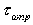is independent of Tnp. If mnp is modeled by (48) the quadratic equation for (Tnp ­ TL)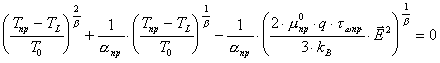(51)

with the physical relevant solution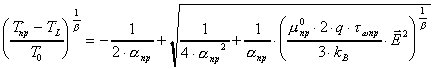(52)

is obtained.

Using (52) in (48) the mobility can be expressed as a function of the electric field:. (53)

Further the limit of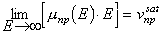(54)

can be determined which reveals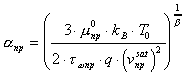. (55)

Under the above made assumptions the HD mobility model (48) corresponds to the following field dependent model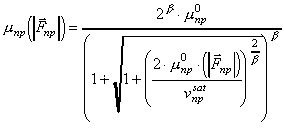. (56)

Using (52) implicitly a carrier temperature for the DD model can be estimated by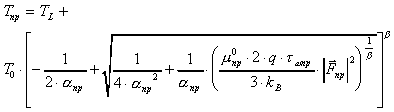. (57)

The carrier temperature revealed from a DD simulation and HD simulation in the channel of a HEMT are compared in Figure  4.3. The maximum carrier temperature obtained for the DD model is about 15 % lower than the maximum carrier temperature obtained with the HD model.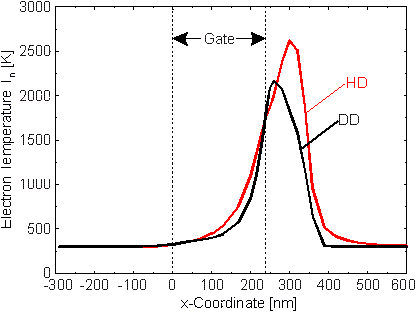Figure 4.3 Carrier temperature along the channel of a HEMT obtained implicitly by DD simulation (bold line) and directly by HD simulation (dashed line).

As shown in (46) and (47) the gradient of the carrier temperature for the driving force is neglected for the DD model. This is different to the driving force of the HD model which reads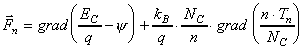(58)

for electrons and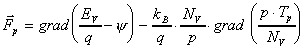(59)

for holes. Taking the gradient of the carrier temperature into account leads to a larger driving force for the HD model compared to the DD model. The difference is shown in  Figure 4.4 for DD and HD simulation again along the channel of a HEMT.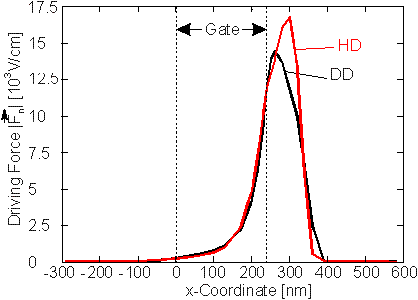Figure 4.4 Driving force along the channel of a HEMT obtained by DD simulation (bold line) and by HD simulation (dashed line).

In addition to the different relations between carrier temperature and driving force for the DD and the HD model another important difference can be observed in Figure 4.3 and  Figure 4.4. The maximum of both the carrier temperature and the driving force is reached for a larger distance from the end of the gate. This is because a finite energy relaxation time is used for the HD model whereas the carriers in the DD model relax instantaneously, thus, a local relation between electric field and carrier velocity exists for the DD model.

As a nonlocal behavior of the carriers is taken into account in the HD model a spatial velocity overshoot can be obtained. The importance and modeling of the spatial velocity overshoot will be discussed further in Section 5.2.

Helmut Brech
1998-03-11